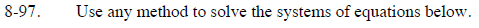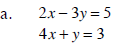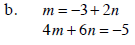### Home > CCA > Chapter 8 > Lesson 8.2.4 > Problem8-97

8-97.
1. Use any method to solve the systems of equations below. Homework Help ✎

1. 2x − 3y = 5
4x + y = 3

2. m = −3 + 2n
4m + 6n = −5(−2, 0.5)